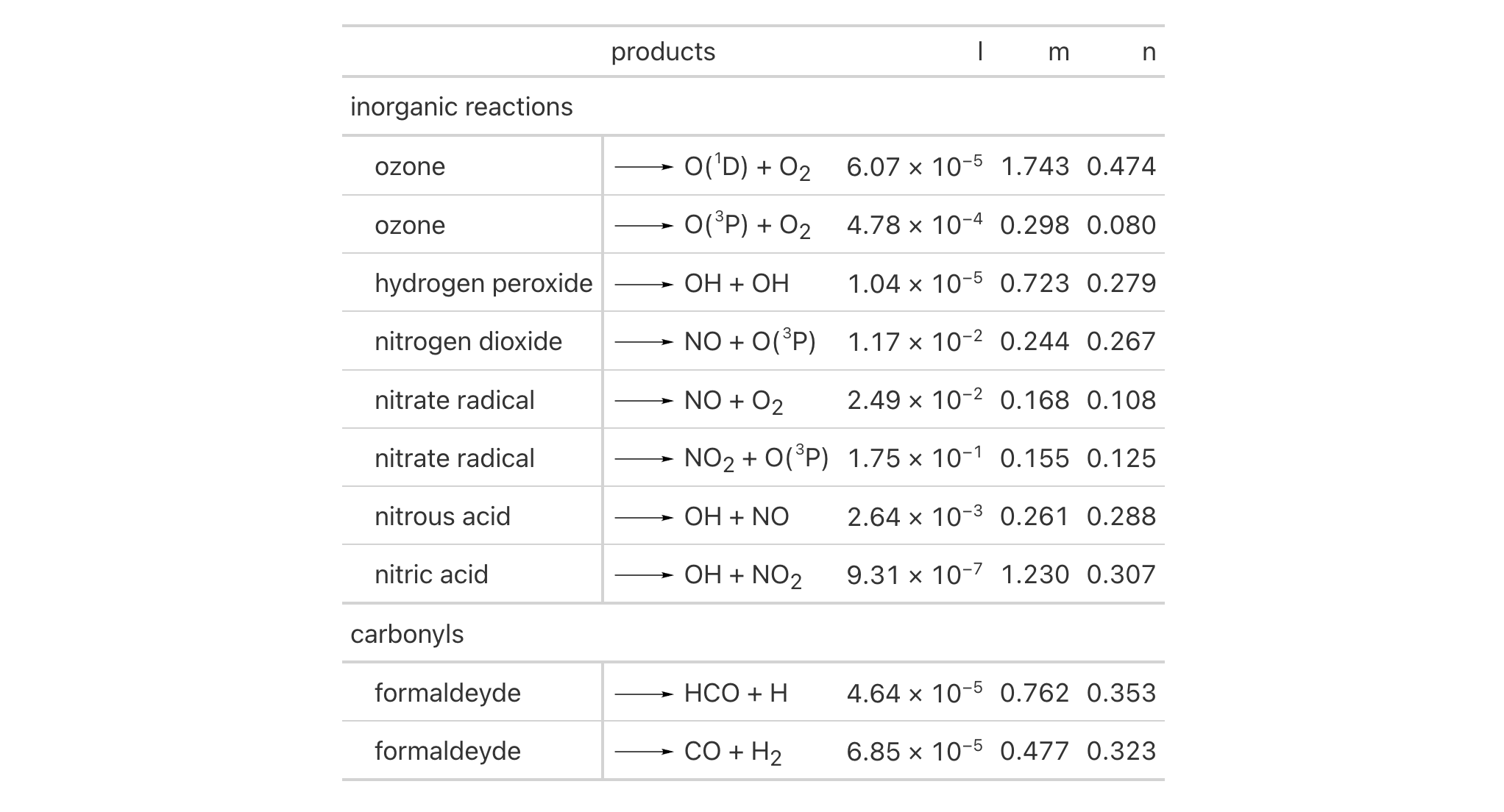Indentation of row labels is an effective way for establishing structure in a table stub. The tab_stub_indent() function allows for fine control over row label indentation through either explicit definition of an indentation level, or, by way of an indentation directive using keywords.

## Usage

tab_stub_indent(data, rows, indent = "increase")

## Arguments

data

A table object that is created using the gt() function.

rows

The rows to consider for the indentation change. Can either be a vector of row captions provided in c(), a vector of row indices, or a helper function focused on selections. The select helper functions are: starts_with(), ends_with(), contains(), matches(), one_of(), and everything().

indent

An indentation directive either as a keyword describing the indentation change or as an explicit integer value for directly setting the indentation level. The keyword "increase" (the default) will increase the indentation level by one; "decrease" will do the same in the reverse direction. The starting indentation level of 0 means no indentation and this values serves as a lower bound. The upper bound for indentation is at level 5.

## Value

An object of class gt_tbl.

## Examples

Use pizzaplace to create a gt table. With tab_stub_indent() we can add indentation to targeted row labels in the stub. Here we target the different pizza sizes and avoid selecting the repeating "All Sizes" row label.

dplyr::bind_rows(
pizzaplace %>%
dplyr::group_by(type, size) %>%
dplyr::summarize(
sold = n(),
income = sum(price),
.groups = "drop_last"
) %>%
dplyr::summarize(
sold = sum(sold),
income = sum(income),
size = "All Sizes",
.groups = "drop"
),
pizzaplace %>%
dplyr::group_by(type, size) %>%
dplyr::summarize(
sold = n(),
income = sum(price),
.groups = "drop"
)
) %>%
gt(rowname_col = "size", groupname_col = "type") %>%
tab_header(title = "Pizzas Sold in 2015") %>%
fmt_number(
columns = sold,
decimals = 0,
use_seps = TRUE
) %>%
fmt_currency(
columns = income,
currency = "USD"
) %>%
tab_options(
summary_row.background.color = "#ACEACE",
row_group.background.color = "#FFEFDB",
row_group.as_column = TRUE
) %>%
tab_stub_indent(
rows = matches("^L|^M|^S|^XL|^XXL"),
indent = 2
) %>%
tab_style(
style = cell_fill(color = "gray95"),
locations = list(
cells_body(rows = matches("^All")),
cells_stub(rows = matches("^All"))
)
)## Function ID

2-6

Other part creation/modification functions: tab_footnote(), tab_header(), tab_options(), tab_row_group(), tab_source_note(), tab_spanner_delim(), tab_spanner(), tab_stubhead(), tab_style()